Courses

# Summary - Friction of Rigid Bodies Mechanical Engineering Notes | EduRev

## Mechanical Engineering : Summary - Friction of Rigid Bodies Mechanical Engineering Notes | EduRev

The document Summary - Friction of Rigid Bodies Mechanical Engineering Notes | EduRev is a part of the Mechanical Engineering Course Engineering Mechanics.
All you need of Mechanical Engineering at this link: Mechanical Engineering

Virtual work

If a body is in equilibrium under the action of a system of forces, the work done is zero since there are no displacements. But if we assume that a body in equilibrium undergoes small imaginary displacements (known as virtual displacements) consistent with the geometrical conditions, imaginary work is said to be done by the system of forces. This imaginary work done is called virtual work.

The body A shown below is in equilibrium under the action of forces. However it assumed that it undergoes an imaginary displacement in the direction of force F.
Displacement in direction of force = Δs cos α Virtual work done = F Δs cos α.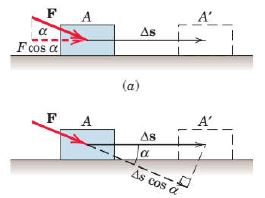Principle of virtual work

If a system of forces acting on a body or a system of bodies is in equilibrium, the total virtual work done by the forces acting on the body or system of bodies is zero for any virtual displacement consistent with geometrical conditions.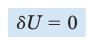For example, consider the free-body diagram of the particle (ball) that rests on the floor. If we “imagine” the ball to be displaced downwards a virtual amount δy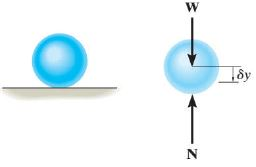Total virtual work done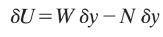Since the system is in equilbrium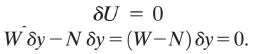Since δy ≠ 0, then  N = W

A. APPLICATION OF THE PRINCIPLE OF VIRTUAL WORK ON BEAMS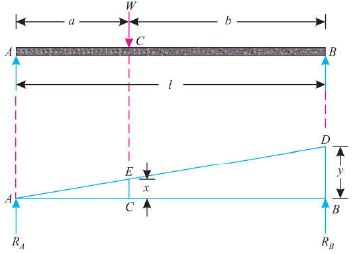Offer running on EduRev: Apply code STAYHOME200 to get INR 200 off on our premium plan EduRev Infinity!

## Engineering Mechanics

37 videos|85 docs|64 tests

,

,

,

,

,

,

,

,

,

,

,

,

,

,

,

,

,

,

,

,

,

;